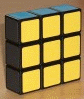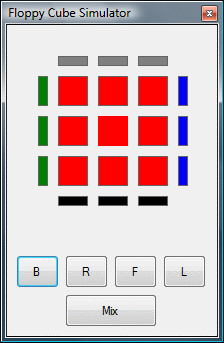# Visual C# GuideFloppy Cube Project - The Problem

## The Floppy CubeA floppy cube is a simple combinatorial puzzle in the style of a Rubik's Cube. It consists of 9 connected cubes or cubies. Groups of 3 cubies can be turned through 180°. The diagram below shows the turns that are possible on the puzzle.Performing one of these turns transforms the state of the puzzle. The two corner cubies swap places and are flipped. The edge cubie is flipped but stays in place.

## The Problem

The problem that this project starts to address can be summarised with the following questions,

• How many unique states can the puzzle be placed in using only combinations of the 4 turns (without rotating the whole puzzle)?
• What is the maximum number of turns required to solve the puzzle?
• What are the combinations of turns required to solve the puzzle from each of these positions?

## Simulation

If you can't lay your hands on the actual puzzle, you can use a software simulation. Here is one made in C# 2008. You use the buttons to make moves on the puzzle. The small rectangles show the colours visible on the sides of the puzzle. You don't get to see the bottom face but shouldn't need to. If the square is red, the one underneath must be yellow and vice versa.